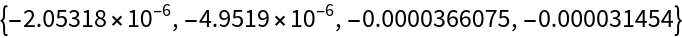Function Repository Resource:

# BlockEntropy

Calculate the joint information entropy of a data matrix

 ResourceFunction["BlockEntropy"][data] gives the joint information entropy of data. ResourceFunction["BlockEntropy"][list,blocksize] computes the entropy after partitioning list by blocksize. ResourceFunction["BlockEntropy"][… , macrofun] groups matrix rows by distinctness of the values macrofun[rowi]. ResourceFunction["BlockEntropy"][… , macrofun,probfun] allows custom conditional probabilities input through probfun. ResourceFunction["BlockEntropy"][k,…] gives the base k joint information entropy.

## Details

ResourceFunction["BlockEntropy"] is similar to Entropy in that it also computes values from a sum of the form -∑piLog[pi].
The entropy measurement starts with grouping rows by Total.
Typically, pi is a frequentist probability of obtaining the ith distinct element by randomly sampling the input list.
ResourceFunction["BlockEntropy"] expects a matrix structure, either of the form data={row1,row2,}, or implicitly as Partition[list,blocksize] ={row1,row2,}.
Additionally, BlockEntropy allows for coarse-graining of rows to macrostates using the function macrofun (default: Total).
Two rows rowj and rowk, with macrostates mj=macrofun[rowj] and mk=macrofun[rowk], are considered distinct if mjmk.
Likewise, two atomistic states dj,x and dk,y are considered distinct if dj,xdk,y.
Let 𝒟 be the set of unique atomistic states and ℳ the set of distinct values in the range of macrofun. The joint entropy is then calculated by a double sum, S=-∑ℙ(dj❘mi)ℙ(mi)Log[ℙ(dj❘mi)ℙ(mi)], where indices i and j range over the elements of ℳ and 𝒟 respectively.
The frequentist probability ℙ(mi) , mi∈ℳ equals the count of rows satisfying mi=macrofun[rowj], divided by the total number of rows.
The conditional probability ℙ(dj❘mi), mi∈ℳ, dj∈𝒟 is not necessarily frequentist, but is often assumed or constructed to be so.
The optional function probfun takes mi∈ℳ as the first argument and blocksize as the second argument. It should return an Association or List of conditional probabilities ℙ(dj❘mi).
When probfun is not set, either "Micro" or "Macro" conditional probabilities can be specified by setting the "Statistics" option.
The default "Micro" statistics obtains 𝒟 by taking a Union over row elements. The conditional probabilities are then calculated as ℙ(dj❘mi)=∑ℙ(djrowk)ℙ(rowk)=∑ℙ(djrowk) /N, where the sum includes every possible rowk written over elements 𝒟 and satisfying mi=macrofun[rowk]. The factor 1/ℙ(rowk)=N equals the Count of such rows, all assumed equiprobable.
Traditional "Macro" statistics require that 𝒟 contains all possible rows of the correct length whose elements are drawn from the complete set of row elements using Tuples. The conditional probabilities are then calculated as ℙ(dj❘mi)=0 if mimacrofun[dj] or if mi=macrofun[dj] as ℙ(dj❘mi)=1 /N, with N equal to the count of atomistic row states dk satisfying mi=macrofun[dk].

## Examples

### Basic Examples (4)

Calculate the BlockEntropy of a binary matrix:

 In:=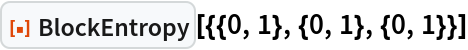Out=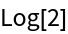The BlockEntropy value does not change by permuting in block:

 In:=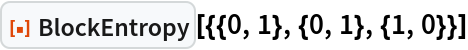Out=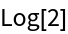Calculate the ternary BlockEntropy of a binary list:

 In:=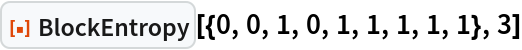Out=Calculate the same value from a matrix input:

 In:=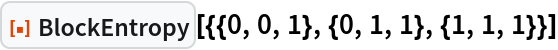Out=The BlockEntropy value does not change by permuting blocks:

 In:=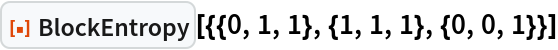Out=Calculate the ternary entropy of a binary list assuming isentropic macrostates:

 In:=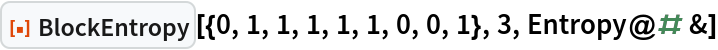Out=Changing the aggregation function can change the BlockEntropy value:

 In:=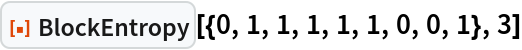Out=Two different aggregation functions can have the same asymptotics:

 In:=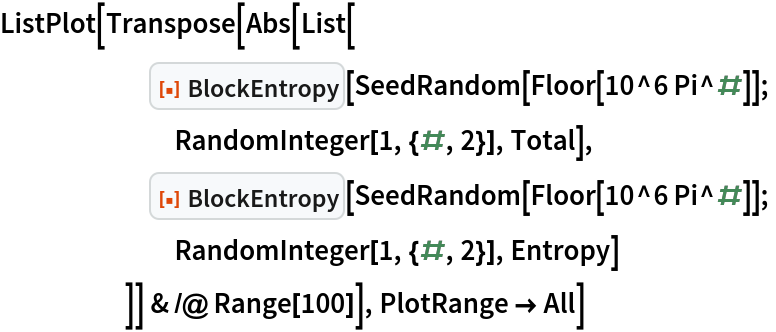Out=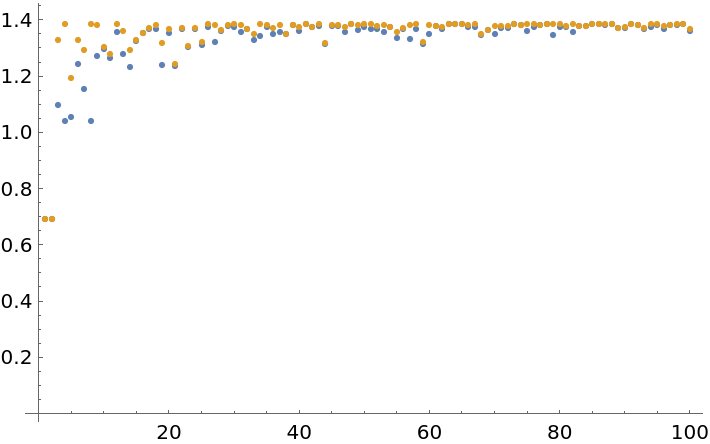### Scope (2)

BlockEntropy accepts lists of arbitrary arity:

 In:=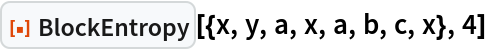Out=Treat each macrostate as equiprobable:

 In:=Out=Compare with more simple means:

 In:=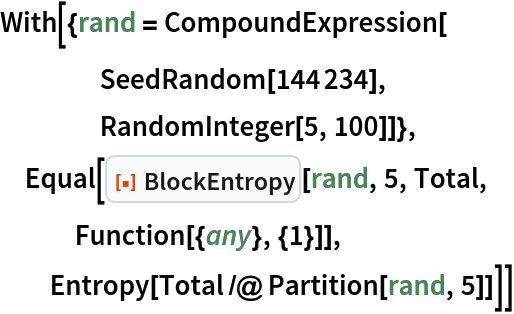Out=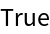### Options (4)

BlockEntropy provides different built-in statistics:

 In:=Out=Setting Statistics to "Macro" can make normal Entropy easier to predict:

 In:=Out=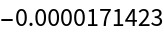Alternative statistics can sometimes have opposite behaviors:

 In:=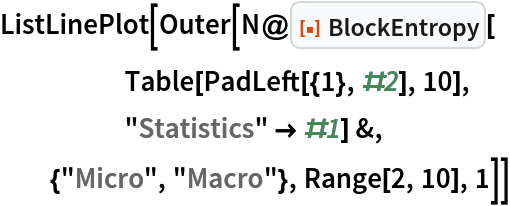Out=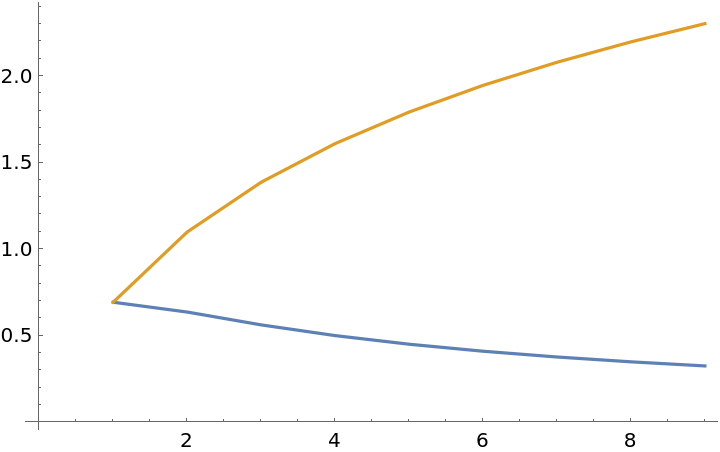Compare with the theoretical result:

 In:=Out=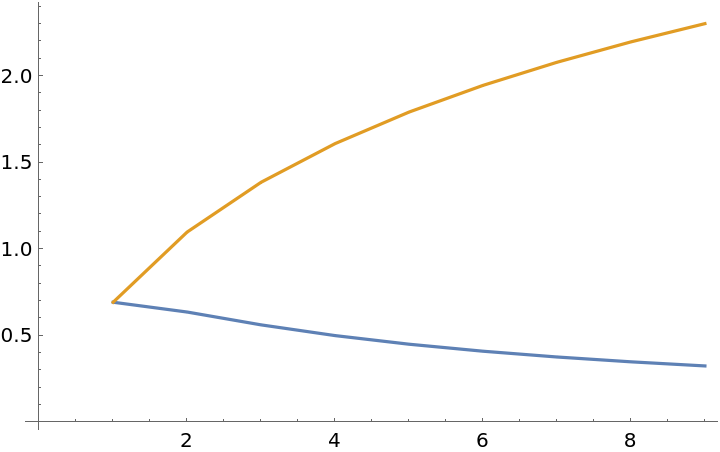### Applications (1)

Measure the entropy time series of a cellular automaton:

 In:=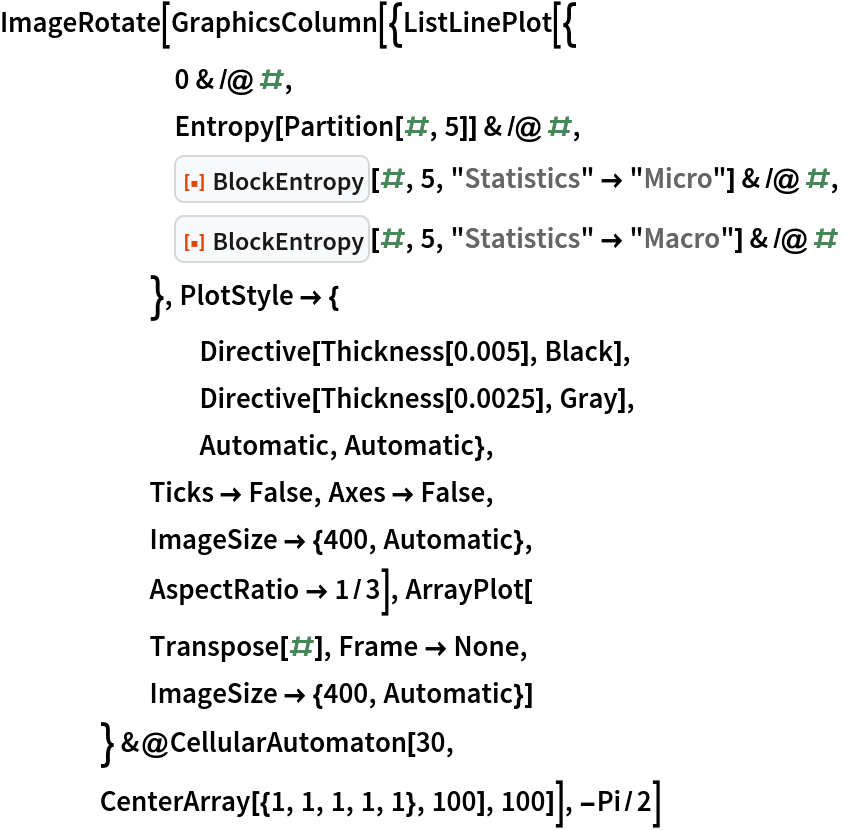Out=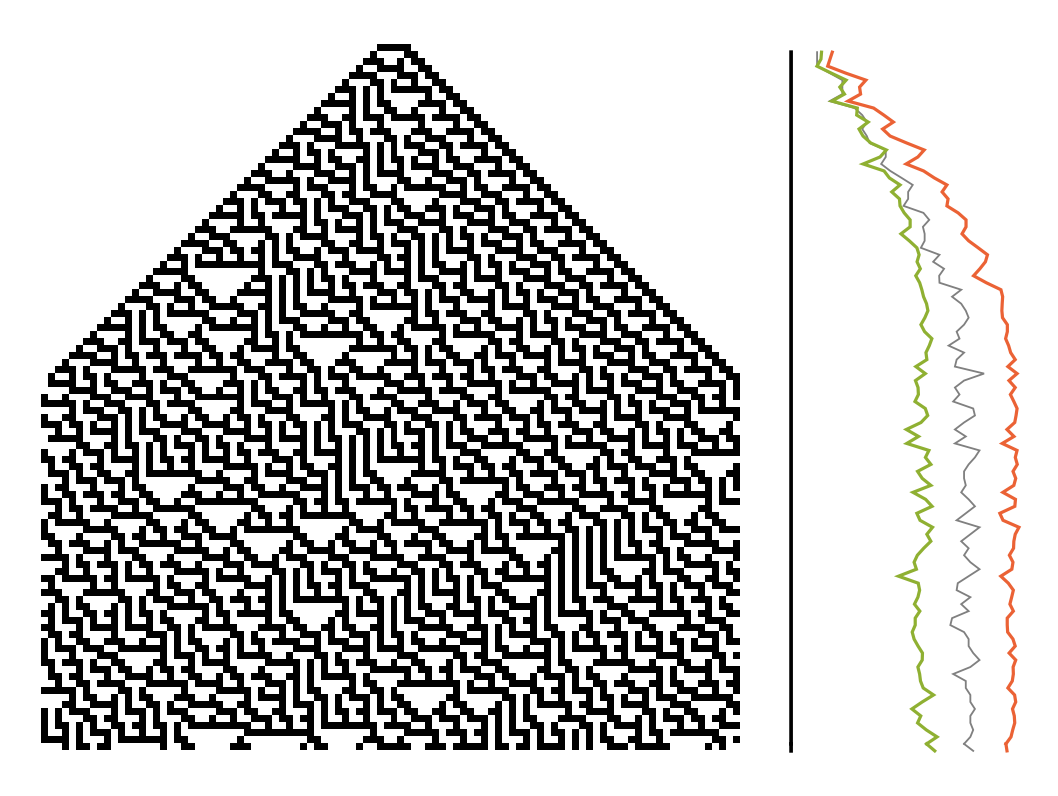### Properties and Relations (3)

The Entropy of a list equals the BlockEntropy of a column matrix with the same elements:

 In:=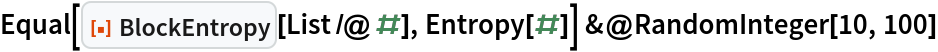Out=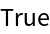BlockEntropy can return the same value as a naive combination of Partition and Entropy:

 In:=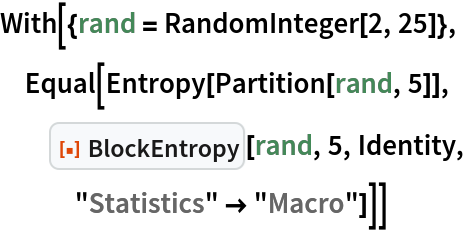Out=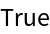The BlockEntropy of a constant density binary matrix equals the Entropy of any row:

 In:=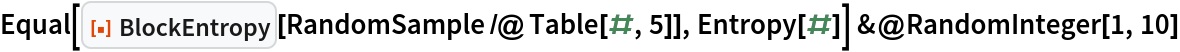Out=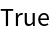But this relation does not hold in general:

 In:=Out=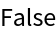### Possible Issues (3)

If an incommensurate block length is chosen, some values will be dropped:

 In:=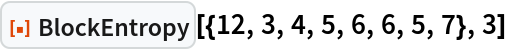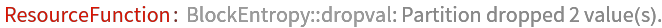Out=The message refers to the underlying behavior of Partition:

 In:=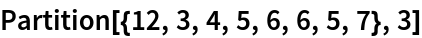Out=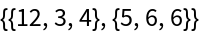Thus the same BlockEntropy value may be computed as:

 In:=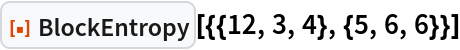Out=### Neat Examples (2)

Classify all length 4 ternary lists according to their binary BlockEntropy:

 In:=Out=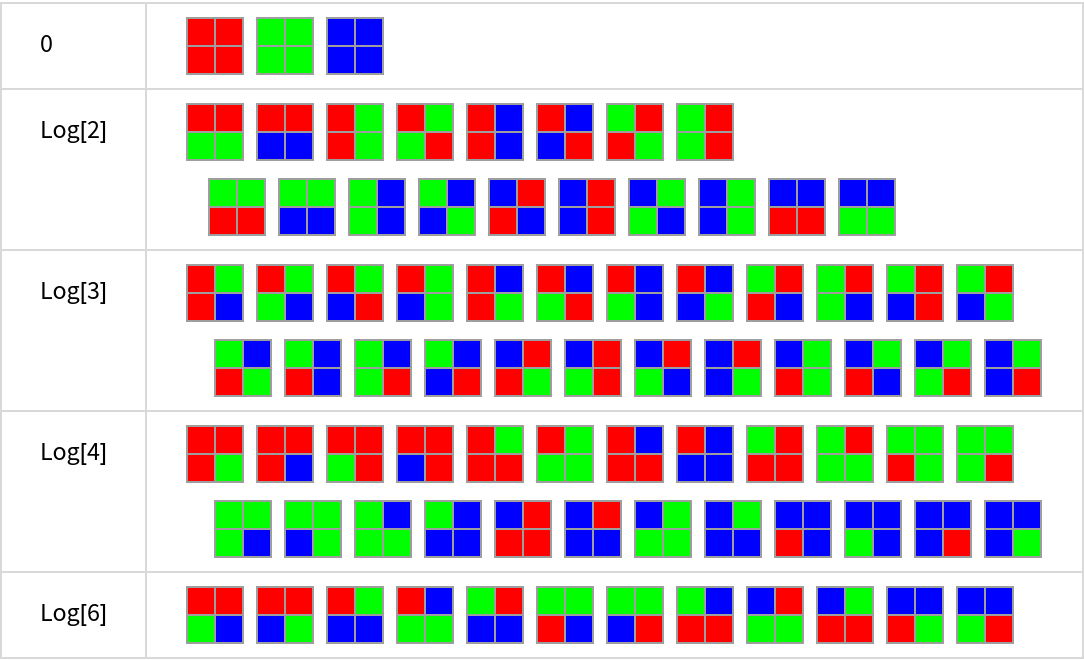Test the randomness of the binary digits of π:

 In:=Out=